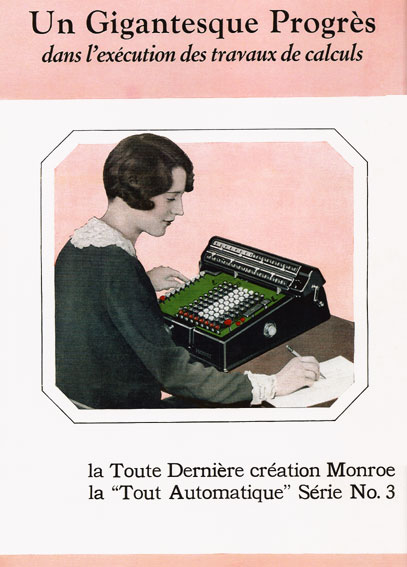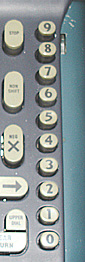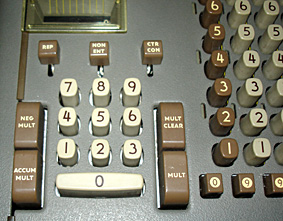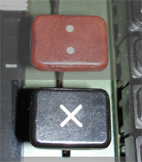The automation of the multiplication

The first machines provided with an electrical motor for the drive are marketed around the year 1910. For simplest of these calculators, the electrical motor replaced simply the crank and did not modify the way to do the calculations.
For these calculators the multiplication was not automatized.

The repetitive multiplication

For the simplest machines, the multiplication is named repetitive. A driving key starts the motor which stops only when the key is released. The operator maintains this key down and counts with the ear (or by supervising the content of the counter) the number of cycles carried out . The key is released when the proper number is achieved.

The automatic multiplicationMost sophisticated of the electric calculators were able to carry out the multiplication automatically. This automatism is more complicated to implement than that of division. Indeed, division being done by successive subtractions it is possible to detect the moment when one makes a subtraction of too, to correct them with an addition cycle and to start a mechanism to automatically shift the carriage in order to pass to the next decimal order.

The multiplication being done by successive additions, it is not possible without a setting in memory of the multiplier to know how many additions must be carried out in each decimal order.
Thus the calculators making the automatic multiplication have a mechanism making it possible to memorize the various figures of the number composing the multiplier.
According to the marks and the models, the multiplier is entered in the memory only once or the figures which compose it are entered one after the other during the calculation. Each one of them is decreased of a unit to each cycle until it becomes equal to zero, indicating that it is necessary to pass to the next decimal order.

Dedicated column for the multiplierA supplementary column of 10 numbered keys is located on a side of the keyboard . When one of this keys is pulled down, the motor starts for a number of cycles corresponding to the figures printed on the keys. When the proper number of cycles is reached, the carriage shifts to the next position and the motor stops. The multiplication consists in entering the multiplicand to the keyboard and pressing sequentially on the keys of the supplementary column corresponding to the figures of the multiplier number starting with the unit one. Most sophisticated of these calculators are equipped with a tabulator allowing to automatically set the carriage on a choosen column , the figures of the multiplier are then typed on the side keyboard in the natural writing order (or the opposite).
Some calculators made it possible to enter the figures of the multiplier the ones after the others without awaiting the completion of the successive cycles of each one of them.

The multiplicator keyboardThis type of calculators are equipped with two keyboards. The main keyboard dedicated to the additions, subtractions, inscription of the multiplicand and a small keyboard intended to record the multiplicator. When the 'multiplication' key is actuated, the computer permanently adds the multiplicand to the accumulator content. At each cycle the multiplier decreases until becoming null, what starts the shift of the carriage to pass to the following decimal order (or the machine stops when the multiplying register is completely emptied).

Multiplier and multiplicand successively entered on the main keyboard

Another method consists in making use of only one keyboard to enter successively the multiplier and the multiplicand. It is the case on some models of the Monroe mark.
The multiplier is firstly set on the keyboard and sent to the memory by depressing the "ENTER MULTIPLIER" key (a dedicated display allows to check the entered number). The multiplicand is then set to the keyboard. The depression of the "CLEAR MULT" starts the multiplication cycles.

Multiplier and multiplicand simultaneously entered on the main keyboard

Some calculators 'Mercedes' have a large keyboard on which one registers in left parts and right-hand side, the multiplicand and the multiplier (the dividend and the divisor). A depressing of the multiplication (division) key starts the operation.

The called 'short cut' multiplication

In order to minimize the number of cycles, a technic known "short cut multiplication" is used in some calculators. This technic consists of multiplying the multiplicand by the complement to 10 of the figures of the multiplier when they lie between 6 and 9 (9=10-1; 8=10-2; 7=10-3; 6=10-4). For example, the depression of key 7 of the side keyboard results in 3 turns in subtraction mode, a left shift of the carriage followed by an addition cycle. On the whole 4 cycles have been done instead of 7 with the standard method. A multiplication by 987 request 4+3+2=9 cycles instead of 24. The average gain for unspecified multipliers is close to 40%. This technic was sometimes used on manual calculators.

If all the figures of the multiplier are recorded before beginning the multiplication, the shortened multiplication can become particularly interesting from the point of view of the time-saver of calculation. For example to multiply by 99999 it is enough to multiply by 10000-1, which corresponds to 2 cycles of calculation instead of 45 in the traditional method.

The two following movies show a comparison of these two technics with a multiplier equal to 999. To see the movies, clic on the picture hereafter.The keys "MULT" "MULT.NEG" and "MULT.ACC".

ThekKey "MULT" gives the result of the product of the two numbers after zeroing the result of the preceding operation. The key "MULT.NEG" does not erase the contents of the accumulator and the result of the multiplication is subtracted to him; for example T - (A X B). The key "MULT.ACC" corresponds to the accumulative multiplication, the product of the two numbers is added with the contents of the accumulator. Then it is possible to easily carry out a sum of products (A X B) + (C X D) + ...